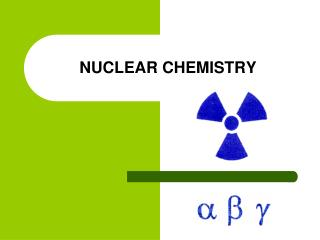Download PresentationNUCLEAR CHEMISTRY

# NUCLEAR CHEMISTRY

Download Presentation## NUCLEAR CHEMISTRY

- - - - - - - - - - - - - - - - - - - - - - - - - - - E N D - - - - - - - - - - - - - - - - - - - - - - - - - - -
##### Presentation Transcript

1. NUCLEAR CHEMISTRY

2. Rates of Decay & Half Life • Radionuclides have different stabilities and decay at different rates.

3. Integrated Rate Equation where… • A = the amt. of decaying sample remaining at some time, t • Ao= the amt. of sample present at the beginning • k = rate constant; different for each radionuclide • t = time

4. Integrated Rate Equation OR… where… • N = # of disintegrations per unit of time; relative activity • No= original activity

5. HALF-LIFE= the amount of time required for half of the original sample to decay

6. HALF-LIFE= the amount of time required for half of the original sample to decay Daughter Parent

7. HALF-LIFE • Half-life = the amount of time required for half of the original sample to decay

8. Example: Cobalt-60 decays with the emission of beta particles and gamma rays, with a half-life of 5.27 years. How much of a 3.42 g of cobalt-60 remains after 30.0 years? How do you solve for A???

9. Take the ANTILOG (10x) of both sides.

10. Example: Cobalt-60 decays with the emission of beta particles and gamma rays, with a half-life of 5.27 years. How much of a 3.42 g of cobalt-60 remains after 30.0 years?

11. Uses of Radionuclides • Radiocarbon dating: the ages of specimens of organic origin can be estimated by measuring the amount of cabon-14 in a sample.

12. Carbon Cycle How does C-14 get into living things???

13. Example: A piece of wood taken from a cave dwelling in New Mexico is found to have a carbon-14 activity (per gram of carbon) only 0.636 times that of wood today. Estimate the age of the wood. (The half-life of carbon-14 is 5730 years.)

14. Example: A piece of wood taken from a cave dwelling in New Mexico is found to have a carbon-14 activity (per gram of carbon) only 0.636 times that of wood today. Estimate the age of the wood. (The half-life of carbon-14 is 5730 years.) t=3744 yrs = 3740 yrs

15. Uses of Radionuclides ***NOTE: Objects older than 50,000 years have too little activity to be dated accurately using carbon dating; instead the following methods are used: • Potassium-40 decays to argon-40: half-life = 1.3 x 109 years • Uranium-238 decays to lead-206: half-life = 4.51 x 109 years

16. Example: A sample of uranium ore is found to contain 4.64 mg of uranium-238 and 1.22 mg of lead-206. Estimate the age of the ore.

17. Example: A sample of uranium ore is found to contain 4.64 mg of uranium-238 and 1.22 mg of lead-206. Estimate the age of the ore.